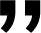# Kelly's Criterion in Forex Trading: Finding the Optimal Bet Size

Article 7 from 7

3 Min

2 Min

2 Min

3 Min

2 Min

3 Min

• ### 7. Kelly's Criterion in Forex Trading: Finding the Optimal Bet Size

3 Min

In forex trading, the ability to determine the appropriate position size is a critical aspect of managing risk and optimizing potential returns. Kelly’s Criterion, a mathematical formula developed by John L. Kelly Jr., provides traders with a systematic approach to calculating the optimal bet size for each trade. This article explores the concept of Kelly’s Criterion in forex trading, its underlying principles, and its application in optimizing trading strategies.

## Understanding Kelly's Criterion

Kelly’s Criterion is a formula that helps traders determine the ideal position size for a trade based on the probability of success and the risk-reward ratio. The formula takes into account the trader’s edge (probability of success) and the reward-to-risk ratio to calculate the optimal percentage of capital to allocate to each trade.

## The Kelly criterion formula has two essential elements:

• Winning probability factor (W): The likelihood that a deal will result in a profit.
• Win/loss ratio (R): This is calculated by dividing the total amount of positive trades by the total amount of negative trades.

## Kelly's Criterion Formulaor, it can be simplify this way:where:

Kelly (%) = Percent of investor’s capital to put into a single trade

W = Historical win percentage of trading system

R = Trader’s historical win/loss ratio

## Principles and Benefits of Kelly's Criterion

1. Maximizing Long-Term Growth: Kelly’s Criterion is designed to maximize the long-term growth rate of a trading account. By allocating capital proportionally to the edge and risk-reward characteristics of each trade, traders aim to achieve optimal account growth over time.
2. Balancing Risk and Reward: The formula strikes a balance between the potential for profit and the risk of loss. It encourages traders to allocate more capital to trades with a higher probability of success and a favorable risk-reward ratio while reducing exposure to riskier trades.
3. Adaptability to Changing Conditions: Kelly’s Criterion adjusts the position size dynamically based on changes in the trader’s edge and risk-reward ratios. This adaptability allows traders to respond to evolving market conditions and adjust their bet sizes accordingly.

## Kelly’s Limitation

There are some people that doubt the Kelly’s Criterion formula. Some economists have argued against the approach despite the fact that it promises to outperform all others in the long term. Their main concern is that an individual’s unique investing limits may come before the goal for the best growth rate.

In actuality, the decision-making capacity of an investor is significantly influenced by their limits, whether or not they are self-imposed. The anticipated utility theory, which states that bets should be sized to maximize expected utility of outcomes, is the traditional alternative.

## Applying Kelly's Criterion in Forex Trading

1. Determine Probability of Success: Based on historical data, backtesting, or technical analysis, estimate the probability of success for a particular trade setup or strategy.
2. Assess Risk-Reward Ratio: Evaluate the potential reward-to-risk ratio for the trade, considering factors such as profit targets and stop-loss levels.
3. Calculate Optimal Bet Size: Plug the probability of success and the reward-to-risk ratio into the Kelly’s Criterion formula to determine the optimal percentage of capital to allocate to the trade.
4. Adjust Position Size: Based on the calculated optimal bet size, adjust the position size for the trade accordingly. Ensure that the position size aligns with the calculated percentage of capital to maximize the potential returns while managing risk.
5. Continuous Evaluation and Monitoring: Regularly assess the performance of your trades and reevaluate the probability of success and risk-reward ratios. Adjust the position sizes accordingly to reflect any changes in market dynamics or trading strategy.

## A Good Kelly’s Ratio

Even while some proponents of the Kelly Criterion will employ the suggested methodology, there are disadvantages to investing a sizable amount of one’s portfolio in a single asset. Even though the Kelly Criterion recommends a higher percentage, an investor should give more than 20% of their bankroll a second thought in the purpose of diversification.

## Conclusion

#### Article Summary